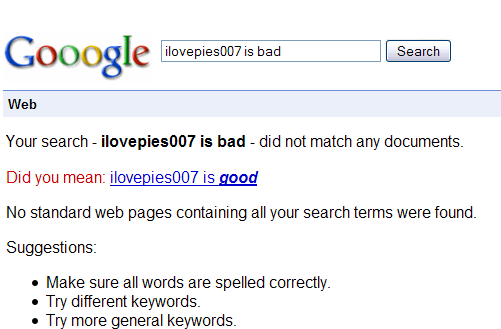# Is this how auto-correct works?Suppose we are given two strings $a$ and $b$. We want to devise a function $f(a,b)$ that tells us how close the two strings are. A reasonable measurement would be to measure how many "edits" operations have to be made to one string in order to change it to the other. There are three possible "edit" operations:

Substitution: Replacing a single character from $a$ so that it matches $b$ costs $1$. If $a=\text{rot}$ and $b=\text{dot}$. Then $f(a,b)=1$.

Insertion: Inserting a single character also costs $1$. Ie, If $a = \text{girl}$ and $b=\text{girls}$, then $f(a,b)=1$.

Deletion: Deleting a single character also costs $1$. Ie. If $a=\text{hour}$ and $b=\text{our}$ then $f(a,b)=1$.

Given $a$ and $b$, compute $f(a,b)$.

×